### Digital Logic Circuits - SYNCHRONOUS SEQUENTIAL CIRCUIT

SYNCHRONOUS SEQUENTIAL CIRCUIT

Introduction

Combinational logic refers to circuits whose output is strictly depended on the present value of the inputs. As soon as inputs are changed, the information about the previous inputs is lost, that is, combinational logics circuits have no memory. In many applications, information regarding input values at a certain instant of time is required at some future time. Although every digital system is likely to have combinational circuits, most systems encountered in practice also include memory elements, which require that the system be described in terms of sequential logic. Circuits whose outputs depends not only on the present input value but also the past input value are known as sequential logic circuits. The mathematical model of a sequential circuit is usually referred to as a sequential machine.

A general block diagram of a sequential circuit is shown below in Figure 1.

Figure 1. Block Diagram of Sequential Circuit.

The diagram consists of combinational circuit to which memory elements are connected to form a feedback path. The memory elements are devices capable of storing binary information within them. The combinational part of the circuit receives two sets of input signals: one is primary (coming from the circuit environment) and secondary (coming from memory elements). The particular combination of secondary input variables at a given time is called the present state of the circuit. The secondary input variables are also know as the state variables.

The block diagram shows that the external outputs in a sequential circuit are a function not only of external inputs but also of the present state of the memory elements. The next state of the memory elements is also a function of external inputs and the present state. Thus a sequential circuit is specified by a time sequence of inputs, outputs, and internal states.

Synchronous and Asynchronous Operation

Sequential circuits are divided into two main types: synchronous and asynchronous. Their classification depends on the timing of their signals.

Synchronous sequential circuits change their states and output values at discrete instants of time, which are specified by the rising and falling edge of a free-running clock signal. The clock signal is generally some form of square wave as shown in Figure 2 below.

Figure 2. Clock Signal

From the diagram you can see that the clock period is the time between successive transitions in the same direction, that is, between two rising or two falling edges. State transitions in synchronous sequential circuits are made to take place at times when the clock is making a transition from 0 to 1 (rising edge) or from 1 to 0 (falling edge). Between successive clock pulses there is no change in the information stored in memory.

The reciprocal of the clock period is referred to as the clock frequency. The clock width is defined as the time during which the value of the clock signal is equal to 1. The ratio of the clock width and clock period is referred to as the duty cycle. A clock signal is said to be active high if the state changes occur at the clock's rising edge or during the clock width. Otherwise, the clock is said to be active low. Synchronous sequential circuits are also known as clocked sequential circuits.

The memory elements used in synchronous sequential circuits are usually flip-flops. These circuits are binary cells capable of storing one bit of information. A flip-flop circuit has two outputs, one for the normal value and one for the complement value of the bit stored in it. Binary information can enter a flip-flop in a variety of ways, a fact which give rise to the different types of flip-flops. For information on the different types of basic flip-flop circuits and their logical properties, see the previous tutorial on flip-flops.

In asynchronous sequential circuits, the transition from one state to another is initiated by the change in the primary inputs; there is no external synchronisation. The memory commonly used in asynchronous sequential circuits are time-delayed devices, usually implemented by feedback among logic gates. Thus, asynchronous sequential circuits may be regarded as combinational circuits with feedback. Because of the feedback among logic gates, asynchronous sequential circuits may, at times, become unstable due to transient conditions. The instability problem imposes many difficulties on the designer. Hence, they are not as commonly used as synchronous systems.

Summary of the Types of Flip-flop Behaviour

Since memory elements in sequential circuits are usually flip-flops, it is worth summarising the behaviour of various flip-flop types before proceeding further.

All flip-flops can be divided into four basic types: SR, JK, D and T. They differ in the number of inputs and in the response invoked by different value of input signals. The four types of flip-flops are defined in Table 1.

Table 1. Flip-flop Types

FLIP-FLOP NAME

FLIP-FLOP SYMBOL

CHARACTERISTIC TABLE

CHARACTERISTIC EQUATION

EXCITATION TABLE

SR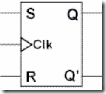S R Q(next) 0 0 Q 0 1 0 1 0 1 1 1 ?

Q(next) = S + R'Q

SR = 0

 Q Q(next) S R 0 0 0 X 0 1 1 0 1 0 0 1 1 1 X 0

JK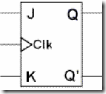J K Q(next) 0 0 Q 0 1 0 1 0 1 1 1 Q'

Q(next) = JQ' + K'Q

 Q Q(next) J K 0 0 0 X 0 1 1 X 1 0 X 1 1 1 X 0

D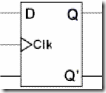D Q(next) 0 0 1 1

Q(next) = D

 Q Q(next) D 0 0 0 0 1 1 1 0 0 1 1 1

T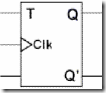T Q(next) 0 Q 1 Q'

Q(next) = TQ' + T'Q

 Q Q(next) T 0 0 0 0 1 1 1 0 1 1 1 0

Each of these flip-flops can be uniquely described by its graphical symbol, its characteristic table, its characteristic equation or excitation table. All flip-flops have output signals Q and Q'.

The characteristic table in the third column of Table 1 defines the state of each flip-flop as a function of its inputs and previous state. Q refers to the present state and Q(next) refers to the next state after the occurrence of the clock pulse. The characteristic table for the RS flip-flop shows that the next state is equal to the present state when both inputs S and R are equal to 0. When R=1, the next clock pulse clears the flip-flop. When S=1, the flip-flop output Q is set to 1. The equation mark (?) for the next state when S and R are both equal to 1 designates an indeterminate next state.

The characteristic table for the JK flip-flop is the same as that of the RS when J and K are replaced by S and R respectively, except for the indeterminate case. When both J and K are equal to 1, the next state is equal to the complement of the present state, that is, Q(next) = Q'.

The next state of the D flip-flop is completely dependent on the input D and independent of the present state.

The next state for the T flip-flop is the same as the present state Q if T=0 and complemented if T=1.

The characteristic table is useful during the analysis of sequential circuits when the value of flip-flop inputs are known and we want to find the value of the flip-flop output Q after the rising edge of the clock signal. As with any other truth table, we can use the map method to derive the characteristic equation for each flip-flop, which are shown in the third column of Table 1.

During the design process we usually know the transition from present state to the next state and wish to find the flip-flop input conditions that will cause the required transition. For this reason we will need a table that lists the required inputs for a given change of state. Such a list is called the excitation table, which is shown in the fourth column of Table 1. There are four possible transitions from present state to the next state. The required input conditions are derived from the information available in the characteristic table. The symbol X in the table represents a "don't care" condition, that is, it does not matter whether the input is 1 or 0.

State Tables and State Diagrams

We have examined a general model for sequential circuits. In this model the effect of all previous inputs on the outputs is represented by a state of the circuit. Thus, the output of the circuit at any time depends upon its current state and the input. These also determine the next state of the circuit. The relationship that exists among the inputs, outputs, present states and next states can be specified by either the state table or the state diagram.

State Table

The state table representation of a sequential circuit consists of three sections labelled present state, next state and output. The present state designates the state of flip-flops before the occurrence of a clock pulse. The next state shows the states of flip-flops after the clock pulse, and the output section lists the value of the output variables during the present state.

State Diagram

In addition to graphical symbols, tables or equations, flip-flops can also be represented graphically by a state diagram. In this diagram, a state is represented by a circle, and the transition between states is indicated by directed lines (or arcs) connecting the circles. An example of a state diagram is shown in Figure 3 below.

Figure 3. State Diagram

The binary number inside each circle identifies the state the circle represents. The directed lines are labelled with two binary numbers separated by a slash (/). The input value that causes the state transition is labelled first. The number after the slash symbol / gives the value of the output. For example, the directed line from state 00 to 01 is labelled 1/0, meaning that, if the sequential circuit is in a present state and the input is 1, then the next state is 01 and the output is 0. If it is in a present state 00 and the input is 0, it will remain in that state. A directed line connecting a circle with itself indicates that no change of state occurs. The state diagram provides exactly the same information as the state table and is obtained directly from the state table.

Example: This example is taken from P. K. Lala, Practical Digital Logic Design and Testing, Prentice Hall, 1996, p.155.

Consider a sequential circuit shown in Figure 4. It has one input x, one output Z and two state variables Q1Q2 (thus having four possible present states 00, 01, 10, 11).

Figure 4. A Sequential Circuit

The behaviour of the circuit is determined by the following Boolean expressions:

 Z = x*Q1 D1 = x' + Q1 D2 = x*Q2' + x'*Q1'

These equations can be used to form the state table. Suppose the present state (i.e. Q1Q2) = 00 and input x = 0. Under these conditions, we get Z = 0, D1 = 1, and D2 = 1. Thus the next state of the circuit D1D2 = 11, and this will be the present state after the clock pulse has been applied. The output of the circuit corresponding to the present state Q1Q2 = 00 and x = 1 is Z = 0. This data is entered into the state table as shown in Table 2.

Present State

 Q1Q2

Next State

 x = 0 x = 1

Output

 x = 0 x = 1

 0 0 0 1 1 0 1 1
 1 1 0 1 1 1 0 0 1 0 1 1 1 0 1 0
 0 0 0 0 0 1 0 1

Table 2. State table for the sequential circuit in Figure 4.

The state diagram for the sequential circuit in Figure 4 is shown in Figure 5.

Figure 5. State Diagram of circuit in Figure 4.

State Diagrams of Various Flip-flops

Table 3 shows the state diagrams of the four types of flip-flops.

 NAME STATE DIAGRAM SR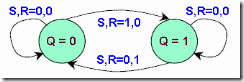JK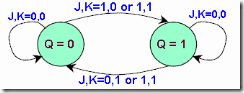D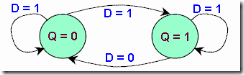T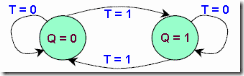All four flip-flops have the same number of states and transitions. Each flip-flop is in the set state when Q=1 and in the reset state when Q=0. Also, each flip-flop can move from one state to another, or it can re-enter the same state. The only difference between the four types lies in the values of input signals that cause these transitions.

A state diagram is a very convenient way to visualise the operation of a flip-flop or even of large sequential components.

1.Circuit board builder is the best editor I have ever used. Bug-free design, excellent simulation. Well done. Https://www.pcbonline.com/blog

2.This comment has been removed by the author.

3.Circuit board builder is the best editor I have ever used. Bug-free design, excellent simulation. Well done.
good idea.
F22 RC Jet
Arrows Rc jet
GSM alarm system
FMS RC Car
security camera
Hobbystar motor
atten soldering gun
PCBA factory
https://www.kingfordpcb.com/